# Fast review:

In this note I want to start a prove chain. This means if you want to continue this chain you should prove something, but you must use the result of the previous approach. For example if the first person proved that $a\cdot b=2A$ in a right triangle and you want to prove that the area of a square is $a^2$, then this is a good approach:

If we cut the square into two right triangles: $A_s=2\cdot A_t;a\cdot b=2A_t;a=b\Rightarrow A_s=2\cfrac{a\cdot a}{2}=a^2$

This can be just a fun note. If you are boring or you want to rest for a few minute, then this is a chance to prove something what you want(and you can). You can prove basic, easy or hard things.

# Rules:

• If you want to share something, what isn't continue this chain, then use the reply button under one of the approaches
• Don't share messages to your friends
• Don't prove something again in this note
• To avoid two approaches at the same time you should reply for the previous approach(use the given text) before you continue this chain
• Don't prove evil things what others can't continue

# $\color{#eeaa00}\text{Temporary rule(s)}$

• $\color{#eeaa00}\text{The first ten approaches should not create a chain}$
• $\color{#eeaa00}\text{You can share not original, but not famous approches}$

# $\color{#D61F06}\text{Current theme(s)}$

• $\color{#D61F06}\text{Number theory}$
• $\color{#D61F06}\text{Algebra}$

# $\color{#3D99F6}\text{Event(s)}$

• $\color{#3D99F6}\text{There has been no events yet(after 10 active members will be the first event)}$

I will change the colored sections sometimes. If you don't want to skip them, then you can subscribe above the comments or if you write an approach, then you will get notifications too. When I change the temporary rule(s) I will notificate you.

$\color{#D61F06}\text{You can also write proofs that do not belong to the given theme(s)}$

# You can:

• Use things that have already been proven before you(you should name which one is that)
• Mention people to join
• Mention somebody to help you continue this chain if you wrote an approach, but you are bad in $\LaTeX$
• You can prove evil things, but then you must continue the chain and prove something easier to help others
• If this is possible you can split up your approach into two approaches

# How to:

## Mention somebody:

@<name>
Example:
@Páll Márton

## Write an approach:

1. Reply for the last approach: I want to continue this chain. If somebody already working on this you should wait.
2. Write your approach. Use this form below:
 1 2 3 4 5 6 # ID: ## ## > 

When you write an approach don't use the reply button. Just write as a comment.

## Use pictures:

The code of your picture will be: ![<text under the picture>](<link to the picture>){: .<position>}

1. Profile->+Note(in the bottom)->Upload an image
2. Copy the code of the picture
• The [] section can be empty
• The <position> can be center,left,right.

## Use $\LaTeX$:

Without spaces:

• \ ( <LaTeX code> \ ) - left aligned
• \ [ <LaTeX code> \ ] - center aligned

If you are bad in $\LaTeX$, then you can use online editors or web pages to learn.

## Use other formatting:

Here you can see how to use links, use bold and italistic styles, use quotes etc.

## The code of the first comment is(without spaces between \ and ( or ) ):

 1 2 3 4 5 6 7 8 # ID: 1 ## Graphically prove that \ (a^2-b^2=(a-b)(a+b)\ ) ## I won't use other formulas from this note Assume that \ (a>b\ ). In this picture below \ (T_?\ ) means territory/area: ![](https://ds055uzetaobb.cloudfront.net/uploads/ZmvJbP3yh4-0.PNG){: .center} In this picture \ (T_1+T_3+T_4=a^2\ ) and \ (T_4=b^2\implies T_1+T_3=a^2-b^2\ ). We know \ (T_1+T_2=(a+b)(a-b)\ ) from the side length of the rectangle. And we know \ (T_2=T_3\ ), because both of them is equal to \ (b(a-b)\ ). Therefore \ (T_1+T_3=T_1+T_2=(a+b)(a-b)\ ). So \ (a^2-b^2=(a-b)(a+b)\ ). > P.S.: If \ (a=b\ ) then \ (a^2-b^2=(a-b)(a+b)=0\ ). And if \ (a

# Good luck!

## Active members:

• If you post an approach, I will give you 5 points, except if your solution is wrong, not complete or confusing.
• If you post an approach about the current theme, then you can get 3 extra points.
• If you write something interesting about the approach (for example ID-3 by David Vreken), then you can get 1 extra points.
 Name Posted approaches Points David Vreken 1 6 Páll Márton 5 Vinayak Srivastava 1 5

If we reach the 10 active members, then I will mark the 3 most active people. And I will mark the winner of the last event too.Note by Páll Márton (no activity)
4 months, 2 weeks ago

This discussion board is a place to discuss our Daily Challenges and the math and science related to those challenges. Explanations are more than just a solution — they should explain the steps and thinking strategies that you used to obtain the solution. Comments should further the discussion of math and science.

When posting on Brilliant:

• Use the emojis to react to an explanation, whether you're congratulating a job well done , or just really confused .
• Ask specific questions about the challenge or the steps in somebody's explanation. Well-posed questions can add a lot to the discussion, but posting "I don't understand!" doesn't help anyone.
• Try to contribute something new to the discussion, whether it is an extension, generalization or other idea related to the challenge.

MarkdownAppears as
*italics* or _italics_ italics
**bold** or __bold__ bold
- bulleted- list
• bulleted
• list
1. numbered2. list
1. numbered
2. list
Note: you must add a full line of space before and after lists for them to show up correctly
paragraph 1paragraph 2

paragraph 1

paragraph 2

[example link](https://brilliant.org)example link
> This is a quote
This is a quote
    # I indented these lines
# 4 spaces, and now they show
# up as a code block.

print "hello world"
# I indented these lines
# 4 spaces, and now they show
# up as a code block.

print "hello world"
MathAppears as
Remember to wrap math in $$ ... $$ or $ ... $ to ensure proper formatting.
2 \times 3 $2 \times 3$
2^{34} $2^{34}$
a_{i-1} $a_{i-1}$
\frac{2}{3} $\frac{2}{3}$
\sqrt{2} $\sqrt{2}$
\sum_{i=1}^3 $\sum_{i=1}^3$
\sin \theta $\sin \theta$
\boxed{123} $\boxed{123}$

Sort by:

- 4 months, 1 week ago

# ID: 1

## I won't use other formulas from this note

Assume that $a>b$. In this picture below $T_?$ means territory/area: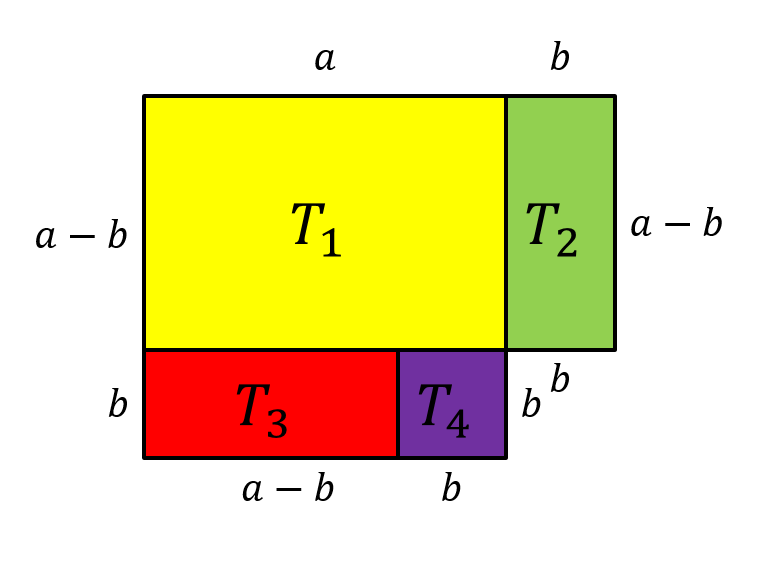In this picture $T_1+T_3+T_4=a^2$ and $T_4=b^2\implies T_1+T_3=a^2-b^2$. We know $T_1+T_2=(a+b)(a-b)$ from the side length of the rectangle. And we know $T_2=T_3$, because both of them is equal to $b(a-b)$. Therefore $T_1+T_3=T_1+T_2=(a+b)(a-b)$. So $a^2-b^2=(a-b)(a+b)$.

P.S.: If $a=b$ then $a^2-b^2=(a-b)(a+b)=0$. And if $a then, then we can multiply by -1. After that we get this: $b^2-a^2=(b-a)(a+b)$. If we swap a and b in the picture we get the same result.

- 4 months, 2 weeks ago

- 4 months, 2 weeks ago

Good, I will try to find something good! Nice note!

- 4 months, 2 weeks ago

Thank you!

- 4 months, 2 weeks ago

I have proof of something else, can I write it in a separate comment?

- 4 months, 1 week ago

Yeah. I will see that :)

- 4 months, 1 week ago

Done!

- 4 months, 1 week ago

# I won't use other formulas from this note

We are usually taught that the proof of the identity ${\sin}^2{\theta}+{\cos}^2{\theta} =1$ is this:

In any right triangle, $O^2+A^2=H^2 \text{ (Where H is the hypotenuse, O is opposite, and A is adjacent.)}$ $\implies \dfrac{O^2}{H^2}+ \dfrac{A^2}{H^2} =1$ $\implies {\sin}^2{\theta}+{\cos}^2{\theta} =1$

However this proof is not complete! What about an angle ${\theta} >90^{\circ}$? It won't form any right triangle!

Here is where our unit circle helps:

In our unit circle, for any angle $360^{\circ}\geq {\theta}\geq 0^{\circ},$ we have the particular point on its circumference representing $\cos{\theta},\sin{\theta}$, as its co-ordinates, I have shown in my diagram: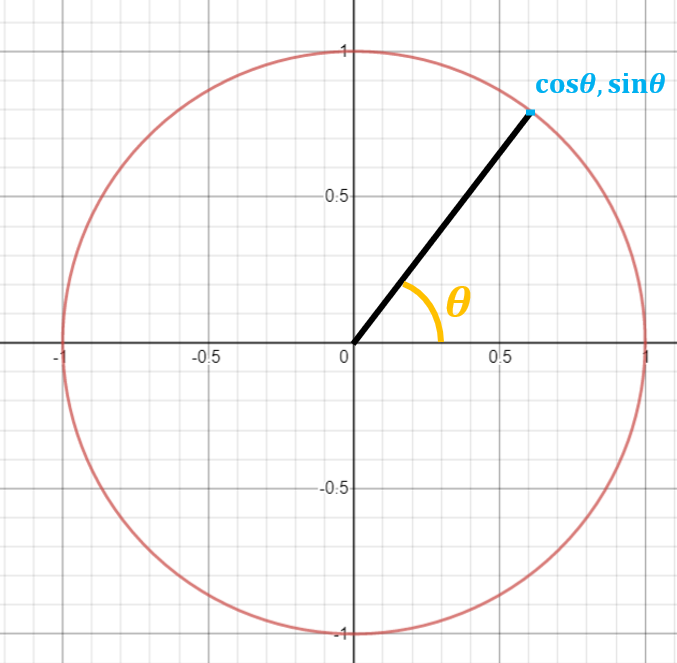But we know that any point on a unit circle satisfies the equation $x^2+y^2=1$

Hence, for any angle $360^{\circ}\geq {\theta}\geq 0^{\circ},$ we get $\Huge{{\sin}^2{\theta}+{\cos}^2{\theta} =1 \blacksquare}$

- 4 months, 1 week ago

Or: $(sin(a))^2+(cos(a))^2=\cfrac{a^2+b^2}{c^2}=1$, where $a^2+b^2=c^2$ :)

- 4 months, 1 week ago

This is the common notation there

- 4 months, 1 week ago

Yes, but I wanted to show that sin=o/h and cos=a/h.

- 4 months, 1 week ago

Please invite more people, it will be fun to prove many things!

- 4 months, 1 week ago

Can you help me in it?

- 4 months, 1 week ago

I have some work of school. Sorry! But, mention few people at a time, it will work.

- 4 months, 1 week ago

Yeah. I have to read 10+ books, but I hate reading :) I will try mention people

- 4 months, 1 week ago

Same with me! :) But I have to write also! :(

- 4 months, 1 week ago

Write a book? :)

- 4 months, 1 week ago

No! Write answers(at least 20 in one chapter)!

- 4 months, 1 week ago

I should write a journal about all of them

- 4 months, 1 week ago

LOL 18 books :) I won't read them. I want to learn english :)

- 4 months, 1 week ago

Now I can't post any approaches with pictures. Can you post an approach about $\sin(90^{\circ}-\alpha)=\cos(\alpha)$? Same thing with cos and tangent, and with 180. I think you can make amazing pictures with unit circles :) I will use them in my approach, but I think we can't skip them.

- 4 months, 1 week ago

Actually, I don't know this formula! I'll search it, and if I understand, I will try.

- 4 months, 1 week ago

# ID: 3

## I won't use other formulas from this note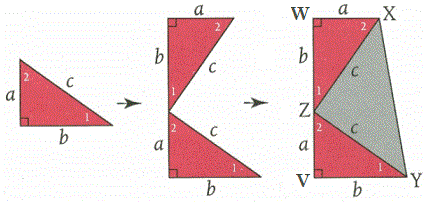Start with a right triangle with legs $a$ and $b$ and hypotenuse $c$. Extend leg $a$ by $b$ units and construct a duplicate right triangle along this extension. Upper leg $a$ is parallel to the original leg $b$ since, in a plane, if a line is perpendicular to each of two lines, then the two lines are parallel. Draw segment $XY$ to close the figure. The resulting quadrilateral is a trapezoid with bases $a$ and $b$ and altitude $a+b$.

The trapezoid is composed of two congruent right triangles and right $\triangle XYZ$. $\triangle XYZ$ is isosceles since two of its sides have length $c$. $\angle XZY$ is a right angle since $\angle 1 + \angle XZY + \angle 2 = 180°$ and $\angle 1 + \angle 2 = 90°$. (The acute angles of a right triangle are complementary.) Therefore, the area of the trapezoid equals the sum of the areas of the three right triangles of which it is composed, two of which are congruent, so that:

\begin{aligned} A_{VWXY} &= A_{\triangle ZVY} + A_{\triangle XWZ} + A_{\triangle XYZ} \\ \frac{1}{2}(a + b)(a + b) &= \frac{1}{2}ab + \frac{1}{2}ab + \frac{1}{2}c^2 \\ (a + b)(a + b) &= ab + ab + c^2 \\ a^2 + 2ab + b^2 &= 2ab + c^2 \\ a^2 + b^2 &= c^2 \end{aligned}

P.S. Written in 1876, James A. Garfield was the only U.S. president to contribute a proof to a mathematical theorem.

- 4 months, 1 week ago

Yeah. In America the presidents are smarter than in Europe. Ferenc Gyurcsány said 11 is the biggest 2-digit prime number :) But the basic approach should be $c^2=a^2+b^2-2ab cos(c);cos(90^{\circ})=0$

- 4 months, 1 week ago

Typically the approach to proving the law of cosines is to use the Pythagorean Theorem, so if you use the law of cosines to prove the Pythagorean Theorem you would have circular reasoning. (Now this almost becomes a philosophical conversation, because it all depends on which premises you want to assume are true.)

- 4 months, 1 week ago

By the way, if you put a slash in front of cos in latex, it won't be italicized (like this: "\cos")

- 4 months, 1 week ago

Wait, Hungary is in Europe! Ugh..............I need to work on my Geography, I thought Hungary was in China or something. I hate my Geo teacher, I think she's a dracanae, trying to kill me.

- 4 months ago

LOL I learned the location of all the countries. And also the states of the United States But I don't remember too much

Wow, that must be hard, it probably is with a dracanae as your teacher.................

- 4 months ago

No, I went to a competition. But I failed on evil geometry. I proved twice this is impossible to solve the problems.

Most of my teacher's(Gym coach is not, coz he likes how much I can swim and my stamina) are monsters, that's why I'm not good at any subject, and that isn't an excuse for my horrible math and science skills(all right it is an excuse, I don't like studying in english letters, they get jumbled up coz of my dyslexia)

- 4 months ago

Hmmm. My teacher who "teached" me sports was released from sports :) BTW he teached me Ukranian language three years long, but he don't have degree to teach them

Released from sports??? @Páll Márton

- 4 months ago

I can't translate it :( When he was young he couldn't play sports.

Good proof Sir! I remember that I once saw a proof in which the triangle is copied 4 times to form a square.

- 4 months, 1 week ago

Yeah. And there are this formula: the length of the two sides of the parallelogram on the square is equal to the sum of the diagonals on the square.

- 4 months, 1 week ago

I haven't heard of anything like this. Can you give a link, or explain yourself?

- 4 months, 1 week ago

@Páll Márton, @David Vreken, @Vinayak Srivastava - Garfield(the mingy, but hilarious cat) was a president of the United States, am I missing something here? And how do you know that Garfield's first name is James?

- 4 months ago

# ID: 4

## I will use this formula from there: ID-3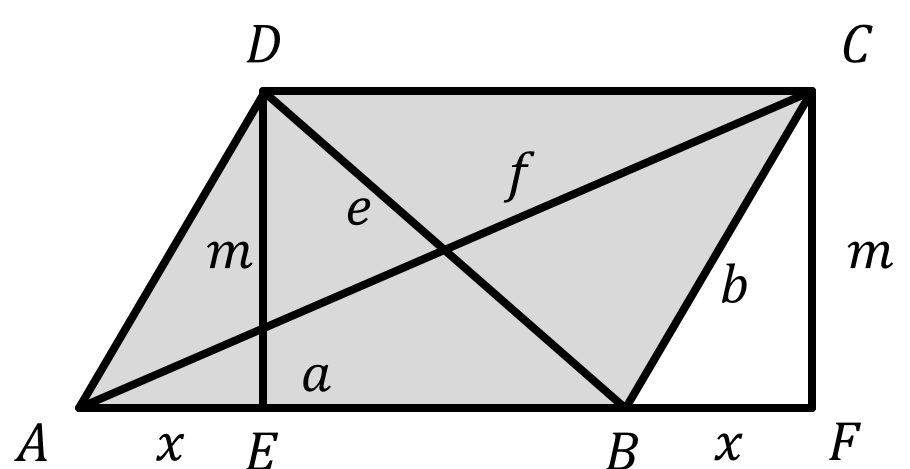From ID-3:

• In $\Delta BFC$: $x^2+m^2=b^2$
• In $\Delta AFC$: $(a+x)^2+m^2=f^2$
• In $\Delta EBD$: $(a-x)^2+m^2=e^2$

The sum of the two last statement: $e^2+f^2=2a^2+\blue{2x^2+2m^2}$. From the first statement: $e^2+f^2=2a^2+2b^2$

You can use this formula too: $c^2=a^2+b^2-2ab\cdot cos(\gamma)$

- 4 months, 1 week ago

Where is point $F$?

- 4 months, 1 week ago

Ohhh. Wait a minute :)

- 4 months, 1 week ago

See now. Thank you!

- 4 months, 1 week ago

Nice proof! I had not heard of this theorem, but seems interesting!

- 4 months, 1 week ago

Yeah. I hate it. My math teacher always use this. Once she allowed me to prove one formula on her lesson, so I started to write a long chain(hence the idea) and I proved this formula

- 4 months, 1 week ago

I will post the next approach :)

- 4 months, 1 week ago

# ID: 5

## I will use these formulas from there: ID-2, ID-3

Assume that $\angle A>\angle B>\angle C$ There are three cases:

## 1.case $\angle A=90^{\circ}$:

From ID-3, this is true, because $cos(90^{\circ})=0$

## 2.case $\angle A<90^{\circ}$: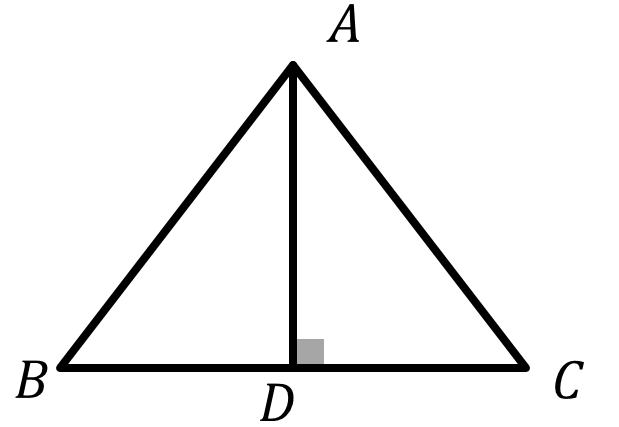$\huge\text{Swap A and B}$ $AD=AB\cdot\;cos(\angle A)$ and $BD=AB\cdot\;sin(\angle A)$
From the 1. case: \begin{aligned} BC^2&=BD^2+DC^2\\ &=BD^2+(AC-AD)^2\\ &=(AB\cdot sin(\angle A))^2+AC^2+(AB\cdot cos(\angle A))^2-2AC\cdot AB\cdot cos(\angle A)\\ &=AB^2(cos^2(\angle A)+sin^2(\angle A))+AC^2-2AC\cdot AB\cdot cos(\angle A) \end{aligned} From ID-2: $BC^2=AB^2+AC^2-2AC\cdot AB\cdot cos(\angle A)$

## 3.case $\angle A>90^{\circ}$:

Same way: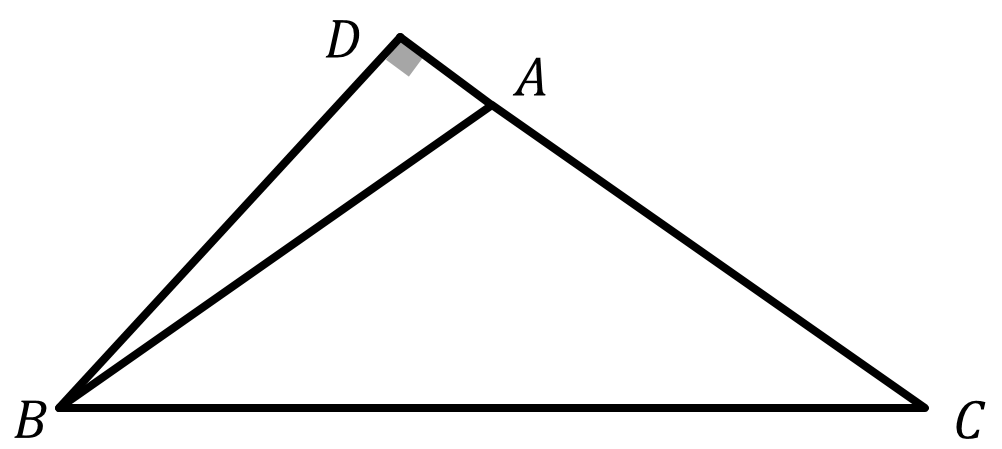$AD=AB\cdot\;cos(\angle BAD)$ and $BD=AB\cdot\;sin(\angle BAD)$
From the 1. case: \begin{aligned} BC^2&=BD^2+DC^2\\ &=BD^2+(AC+AD)^2\\ &=(AB\cdot sin(\angle BAD))^2+AC^2+(AB\cdot cos(\angle BAD))^2+2AC\cdot AB\cdot cos(\angle BAD)\\ &=AB^2(cos^2(\angle BAD)+sin^2(\angle BAD))+AC^2+2AC\cdot AB\cdot cos(\angle BAD) \end{aligned} From ID-2: $BC^2=AB^2+AC^2+2AC\cdot AB\cdot cos(\angle BAD)$, but $cos(180^{\circ}-\alpha)=-cos(\alpha)$. So $BC^2=AB^2+AC^2-2AC\cdot AB\cdot cos(\angle A)$

- 4 months, 1 week ago

I will go to my grandmother, so I won't post approaches in the next couple of days with pictures. Now I will upload an image, to my next approach :) And I will insert it.

- 4 months, 1 week ago

And see the actual theme :)

- 4 months, 1 week ago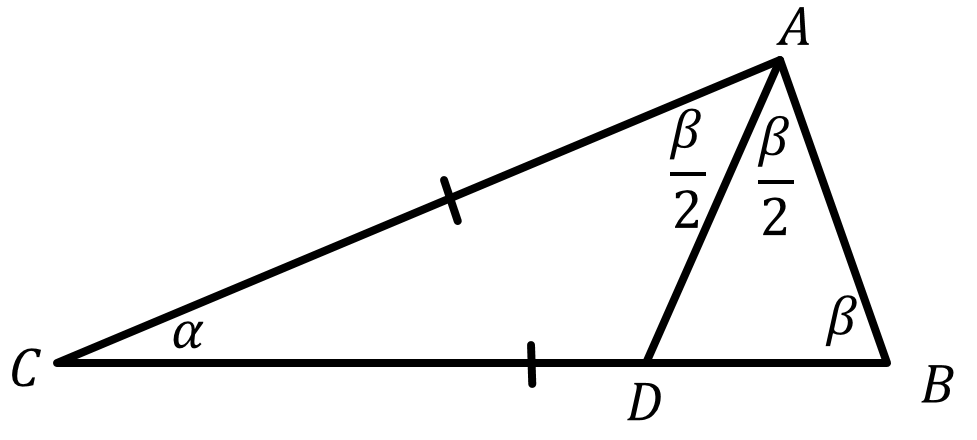I will prove these things:

• $sin(\cfrac{\alpha}{2})=\pm\sqrt{\cfrac{1-cos(\alpha)}{2}}$
• same thing with cos, tan, cot
• $1-tan^2(\alpha)=\cfrac{cos(2\alpha)}{cos^2(\alpha)}$
• same thing with cot

Sorry if there are typos!

- 4 months, 1 week ago

And $cos(\alpha)=cos^2(\alpha /2)-sin^2(\alpha /2)$

- 4 months, 1 week ago

For case 2 I don't think your letters match with your picture (specifically $BC^2 = BD^2 + DC^2$)

- 4 months, 1 week ago

Sorry. Swap A and B :) I will edit it, when I can

- 4 months, 1 week ago

that should do it!

- 4 months, 1 week ago

# ID:6

## Prove these formulas:

• $\sin(\alpha\pm360^{\circ}\cdot k)=\sin(\alpha)$ and $\cos(\alpha\pm360^{\circ}\cdot k)=\cos(\alpha)$, where $k$ is an integer
• $\sin(180^{\circ}-\alpha)=\sin(\alpha)$ and $\cos(180^{\circ}-\alpha)=-\cos(\alpha)$
• $\sin(90^{\circ}-\alpha)=\cos(\alpha)$ and $\cos(90^{\circ}-\alpha)=\sin(\alpha)$
• $\tan(\alpha)=\cfrac{\sin(\alpha)}{\cos(\alpha)}$

## I won't use other formulas from there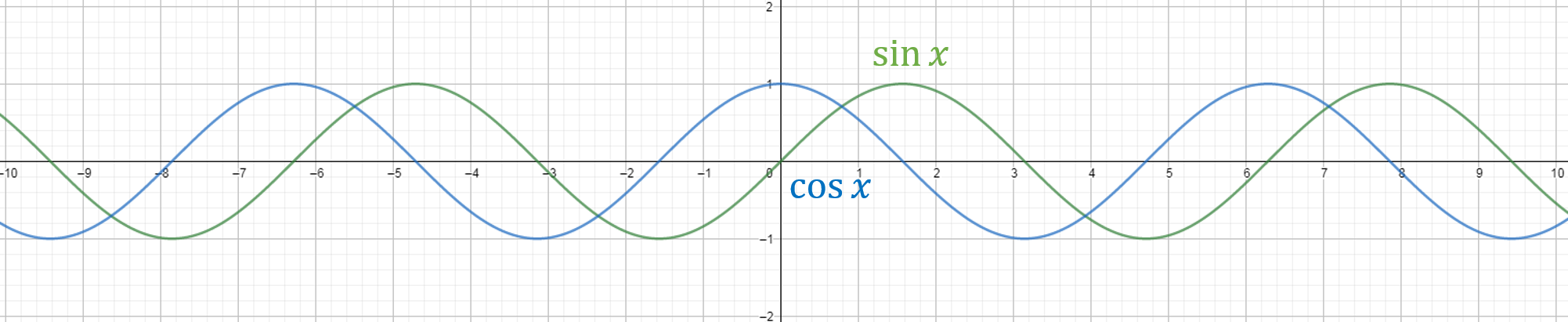In this picture we can see the $\sin(x)$ and $\cos(x)$ functions. We should calculate in radian. This means:

$2\pi=360^{\circ}$, $\pi=180^{\circ}$, $\cfrac{\pi}{2}=90^{\circ}$ We can see if we shift the functions by $2\pi$, then we get the same functions. Same way if we shift these functions by $4\pi,6\pi,8\pi,10\pi$, then we get the same functions.

From the unit circle: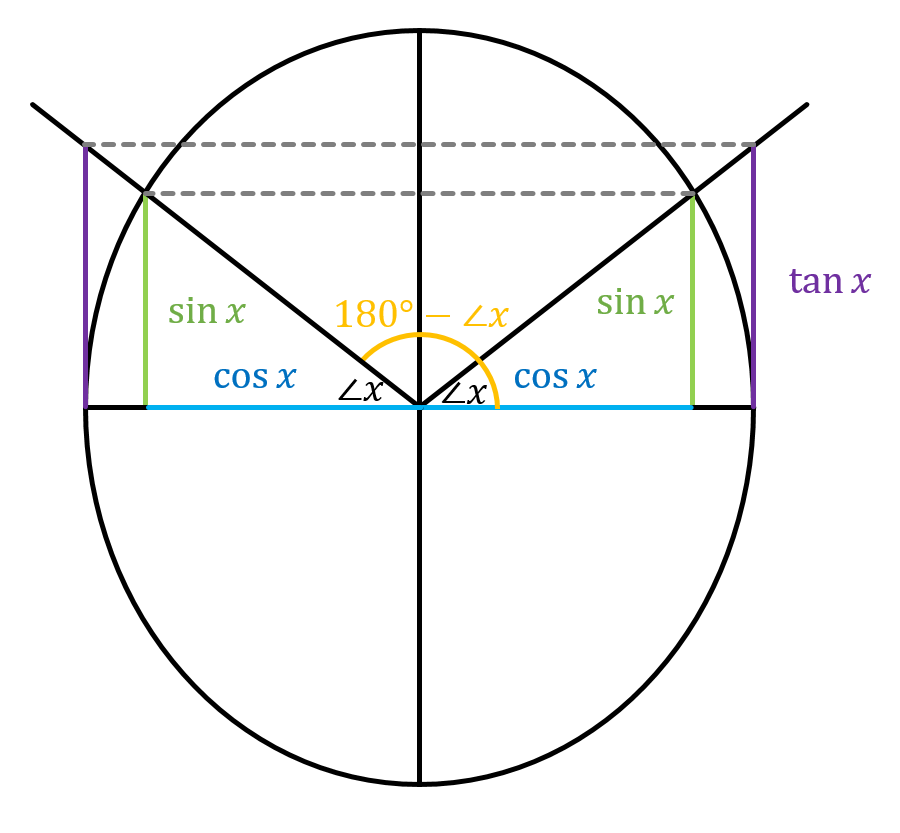$\sin(180^{\circ}-\alpha)=\sin(\alpha)$ and $\cos(180^{\circ}-\alpha)=-\cos(\alpha)$

If $\alpha>180^{\circ}$, then you should mirror the image, and you will get the same result.

From interpretation of $\sin,\cos$: $\sin(\alpha)=\cfrac{a}{c},\cos(\beta)=\cfrac{a}{c},\beta=90^{\circ}-\alpha\implies\cos(90^{\circ}-\alpha)=\sin(\alpha)$. Same way $\sin(90^{\circ}-\alpha)=\cos(\alpha)$ .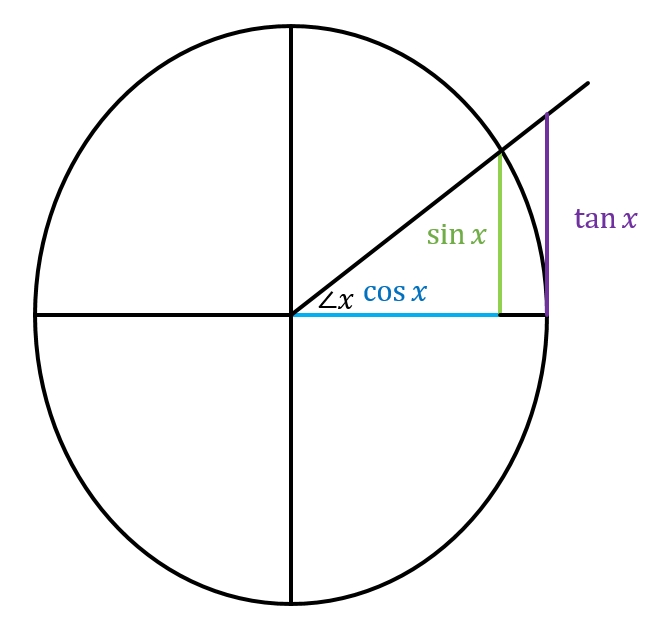From similarity: $\cfrac{\sin(x)}{\cos(x)}=\cfrac{\tan(x)}{1}$

- 4 months, 1 week ago

# ID:7

## Prove these formulas:

• $\sin(\cfrac{\alpha}{2})=\sqrt{\cfrac{1-\cos(\alpha)}{2}}$
• $\cos(\alpha)=\sqrt{\cfrac{1+ \cos(\cfrac{\alpha}{2})}{2}}$
• $\tan(\cfrac{\alpha}{2})=\sqrt{\cfrac{1-\sin(\alpha)}{1+\cos(\alpha)}}$
• $\cot(\cfrac{\alpha}{2})=\sqrt{\cfrac{1+\cos(\alpha)}{1-\sin(\alpha)}}$
• $\cos(2\alpha)=\cos^2(\alpha)-\sin^2(\alpha)$
• $1-\tan^2(\alpha)=\cfrac{\cos(2\alpha)}{\cos^2(\alpha)}$
• $1-\cot^2{\alpha}=-\cfrac{\cos(2\alpha)}{\sin^2(\alpha)}$

## I will use these formulas: ID-5, ID-6a) In this picture AC=BC=x and BD=z From ID-5: \begin{aligned} AD^2&=x^2+(x-z)^2-2x(x-z)\cdot\cos(\alpha)\\ AD^2&=z^2+AB^2-2z\cdot AB\cdot\cos(\beta)\\ AB^2&=x^2+x^2-2x\cdot x \cdot \cos(\alpha)=x^2(2-2\cos(\alpha)) \end{aligned} The left side of the first and the second equations are the same, and we can substitute the third equation to the second, so we can get this long equation: $x^2+(x-z)^2-2x(x-z)\cos(\alpha)=x^2+x^2-2x^2\cdot\cos(\alpha)+z^2-2zx\sqrt{2-2\cos(\alpha)}\cdot\cos(\beta)$ $\blue{x^2+x^2+z^2}-2xz+\blue{2x^2\cdot\cos(\alpha)}+2xz\cdot\cos(\alpha)=\blue{x^2+x^2-2x^2\cdot\cos(\alpha)+z^2}-2zx\sqrt{2-2\cdot\cos(\alpha)}\cdot\cos(\beta)$ We can simplify that. We can substract from both sides the blue section and after that we can divide both sides by $-2xz$: $1-\cos(\alpha)=\sqrt{2-2\cdot\cos(\alpha)}\cdot\cos(\beta)$ \begin{aligned} \cos(\beta)&=\cfrac{1-\cos(\alpha)}{\sqrt{2-2\cos(\alpha)}}\\ &=\cfrac{1-\cos(\alpha)}{\sqrt{2}\sqrt{1-1\cos(\alpha)}}\\ &=\cfrac{\sqrt{1-\cos(\alpha)}}{\sqrt{2}}\\ &= \sqrt{\cfrac{1-\cos(\alpha)}{2}} \end{aligned} We know these things:

• $\beta=\cfrac{180^{\circ}-\alpha}{2}=90^{\circ}-\cfrac{\alpha}{2}$
• $\sin(90^{\circ}-\alpha)=\cos(\alpha)$ $\sin(\cfrac{\alpha}{2})=\sqrt{\cfrac{1-\cos(\alpha)}{2}}$

b) From ID-2: $\sin^2(\alpha)+\cos^2(\alpha)=1$ From ID-7a: \begin{aligned} \cfrac{1-\cos(\cfrac{\alpha}{2})}{2}+cos^2(\alpha)&=1\\ 1- \cos(\cfrac{\alpha}{2})+2\cos^2(\alpha)&=2\\ \cos^2(\alpha)&=\cfrac{1+ \cos(\cfrac{\alpha}{2})}{2}\\ \cos(\alpha)&=\sqrt{\cfrac{1+ \cos(\cfrac{\alpha}{2})}{2}} \end{aligned}

c) From ID-7a and ID-7b: \begin{aligned} \tan(\cfrac{\alpha}{2})&=\cfrac{\sin(\cfrac{\alpha}{2})}{\cos(\cfrac{\alpha}{2})}\\ &=\cfrac{\sqrt{\cfrac{1-\cos(\alpha)}{\cancel{2}}}}{\sqrt{\cfrac{1+\cos(\alpha)}{\cancel{2}}}}\\ &=\sqrt{\cfrac{1-\sin(\alpha)}{1+\cos(\alpha)}} \end{aligned}

d) $\cfrac{1}{\tan(\alpha)}=\cot(\alpha)$. So from ID-7c this is true.

e) From ID-7b: \begin{aligned} \cos(2\alpha)&=2\cos^2(\alpha)-\blue{1}\\ &=2\cos^2(\alpha)-(\sin^2(\alpha)+\cos^2(\alpha))\\ &=\cos^2(\alpha)-\sin^2(\alpha) \end{aligned}

f) \begin{aligned} 1-\tan^2(\alpha)&=1-\cfrac{\sin^2(\alpha)}{\cos^2(\alpha)}\\ &=1- \cfrac{\cfrac{1-\cos(2\alpha)}{2}}{\cos^2(\alpha)}\\ &=\cfrac{ \cos^2(\alpha)-\cfrac{1-\cos(2\alpha)}{2}}{\cos^2(\alpha)}\\ &=-\cfrac{1-2\cos^2(\alpha)-\cos(2\alpha)}{2\cos^2(\alpha)}\\ &=-\cfrac{2-4\cos^2(\alpha)}{2\cos^2(\alpha)}\\ &=\cfrac{2\cos^2(\alpha)-1}{\cos^2(\alpha)}\\ &=\cfrac{\cos(2\alpha)}{\cos^2(\alpha)} \end{aligned}

g) \begin{aligned} 1-\cot^2{\alpha}&=\cfrac{\sin^2(\alpha)-\cfrac{1+\cos(2\alpha)}{2}}{\sin^2(\alpha)}\\ &=\cfrac{ \cfrac{1-\cos(2\alpha)}{2}- \cfrac{1+\cos(2\alpha)}{2}}{\sin^2(\alpha)}\\ &=\cfrac{ -\cos(2\alpha)-\cos(2\alpha)}{2\sin^2(\alpha)}\\ &=-\cfrac{\cos(2\alpha)}{\sin^2(\alpha)} \end{aligned}

- 4 months, 1 week ago

I'm working on this yet :) I should prove that, when $\alpha>180^{\circ}$.

- 4 months, 1 week ago

# ID:8

## I will not use any formulas from other proofs

This is a proof by contradiction, so let's assume that $\sqrt{2}$ is rational.

If so, then it can be expressed in an equation as $\dfrac{p}{q}$ where $p$ and $q$ are co-primes - \begin{aligned} \sqrt{2} &= \dfrac{p}{q} \\ 2 &= \dfrac{p^{2}}{q^{2}} \\ 2p^{2} &= q^{2} \end{aligned}

By this simplification, we know that $q^{2}$ even, because it is $2$ multiplied by some other number. So $q$ must also be an even number. That means $q$ can be represented as $(2m)$.

Back to the equation, if we use the new value of $q$ and simplify further - \begin{aligned} 2p^{2} &= q^{2} \\ 2p^{2} &= (2m)^{2} \\ 2p^{2} &= 4m^{2} \\ p^{2} &= 2m^{2} \end{aligned}

As $p$ can also be represented like this, $p$ must also be even.

This creates a contradiction, as we had earlier said that $p$ and $q$ were co-primes, but now we say they are both even, which means they have a common divisor of $2$. Since this creates a contradiction, our original assumption must have been wrong...

$\text{Q.E.D - } \sqrt{2} \text{ is irrational!}$

This was originally proved by the Greek Mathematician and Father of Geometry, Euclid of Alexandria

@Páll Márton (no activity) - Thoughts? Also, do I have to wait till ID:10 to start chains?

- 4 weeks, 1 day ago

# ID:9

## I will not use any formulas from other proofs

Let's cut a circle into $8$ equal sectors and arrange them as shown in the image below -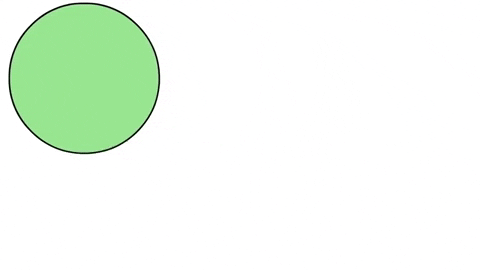Image credits - https://medium.com

As you can see, it forms a figure that looks very close to a parallelogram that is going towards becoming a rectangle. The next two images show what happens when we take even smaller arcs.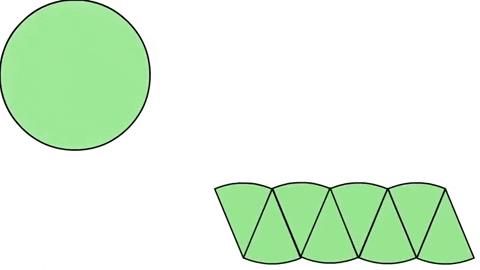Image credits - https://medium.com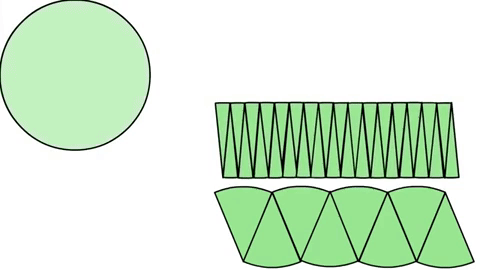Image credits - https://medium.com

In the last image, you see an figure that is almost a rectangle. TO get a perfect rectangle, we would need infinitesimally small sectors, but we can evaluate the area of the circle using this figure, as we know the formula for the area of a rectangle - $A = bh$

The height is easy to find, as it is just the radius. The base, if you look closely at the next image, is actually half of the circumference or half of $2 \pi r$ or $\pi r$Image credits - https://medium.com

Thus, the formula for the area of a circle $\pi r \times r = \boxed{\pi r^{2}}$

- 4 weeks ago

Excellent animations!

- 4 weeks ago

@David Vreken Quite true. How do they even do that?

- 4 weeks ago

Its a mystery, but I'm sure our buddy Mahdi Raza could do much better.

- 4 weeks ago

mmmmmmmmmmmmm!!!!!

- 4 weeks ago

@Percy Jackson1-2 more mins untill daily challenge

- 4 weeks ago

Yess!!!!!

- 4 weeks ago

@NSCS 747 yea for Australian timing right?

- 4 weeks ago

um yea

- 4 weeks ago

K that's good! I have recently learned Aussie slang such as dead horse! Even though I am a migrant Australia who has stayed in the land of Oz for 7 years!!!!!

- 4 weeks ago

i live in NSW sydney so its 10:02 right now

- 4 weeks ago

@NSCS 747 I live in Victoria. Why do so many people live in NSW?!!

- 4 weeks ago

i was born here so i just like it here i havnt been to any other state or city except for newcastle and canberra

- 4 weeks ago

Ah I see I have only stayed in Victoria since I cam to Australia.

- 4 weeks ago

how easy is icas for you?

- 4 weeks ago

Like moderately easy. Got High distinction for MATH ONLY< ONLY!!!!

- 4 weeks ago

for me i always get credit lol and for some reason i always get 81% for maths like always even two part exams part a and part b i get 81%

- 4 weeks ago

oh wait cus the mean is 81% frik im dumb

- 4 weeks ago

Yes! I found them on medium.com, so I image credited each of them under the pics.

- 4 weeks ago

# ID:10

## Algebraic Proof of following Trig Identities

• a) $\sin(90- \beta) = \cos(\beta)$

• b) $\cos(90-\beta) = \sin(\beta)$

## I will not use any formulas from other proofs

a) and b) can be proven using the sine and cosine angle subtraction formulas

• $\cos(\alpha - \beta) = \cos(\alpha)\cos(\beta) + \sin(\alpha)\sin(\beta)$

• $\sin(\alpha - \beta) = \sin(\alpha)\cos(\beta) - \cos(\alpha)\sin(\beta)$

a) $\sin(90- \beta) = \cos(\beta)$

Using the above formula, substituting $\alpha$ with $90$,

\begin{aligned} \sin(90- \beta) &= \sin(90)\cos(\beta) - \cos(90)\sin(\beta) \\ \sin(90- \beta) &= 1 \times\cos(\beta) - 0 \times\sin(\beta) \\ \color{#3D99F6}\sin(90- \beta) \color{#3D99F6} &= \color{#3D99F6}\cos(\beta) \end{aligned}

b) Using the above formula again, substituting $\alpha$ with $90$,

\begin{aligned} \cos(90- \beta) &= \cos(90)\cos(\beta) + \sin(90)\sin(\beta) \\ \cos(90- \beta) &= 0 \times \cos(\beta) + 1 \times \sin(\beta) \\ \color{#3D99F6}\cos(90- \beta) \color{#3D99F6} &= \color{#3D99F6}\sin(\beta)\end{aligned}

@Páll Márton (no activity) - We have reached ID:10!!! Can we start the chain proofs now?

- 4 weeks ago

# ID:11

## Not using other identities

Construct an isosceles triangle $\triangle ABC$.
In isosceles$\triangle ABC$, $BC$ is the base. Construct the median $AM$ where $M$ is the midpoint of $BC$.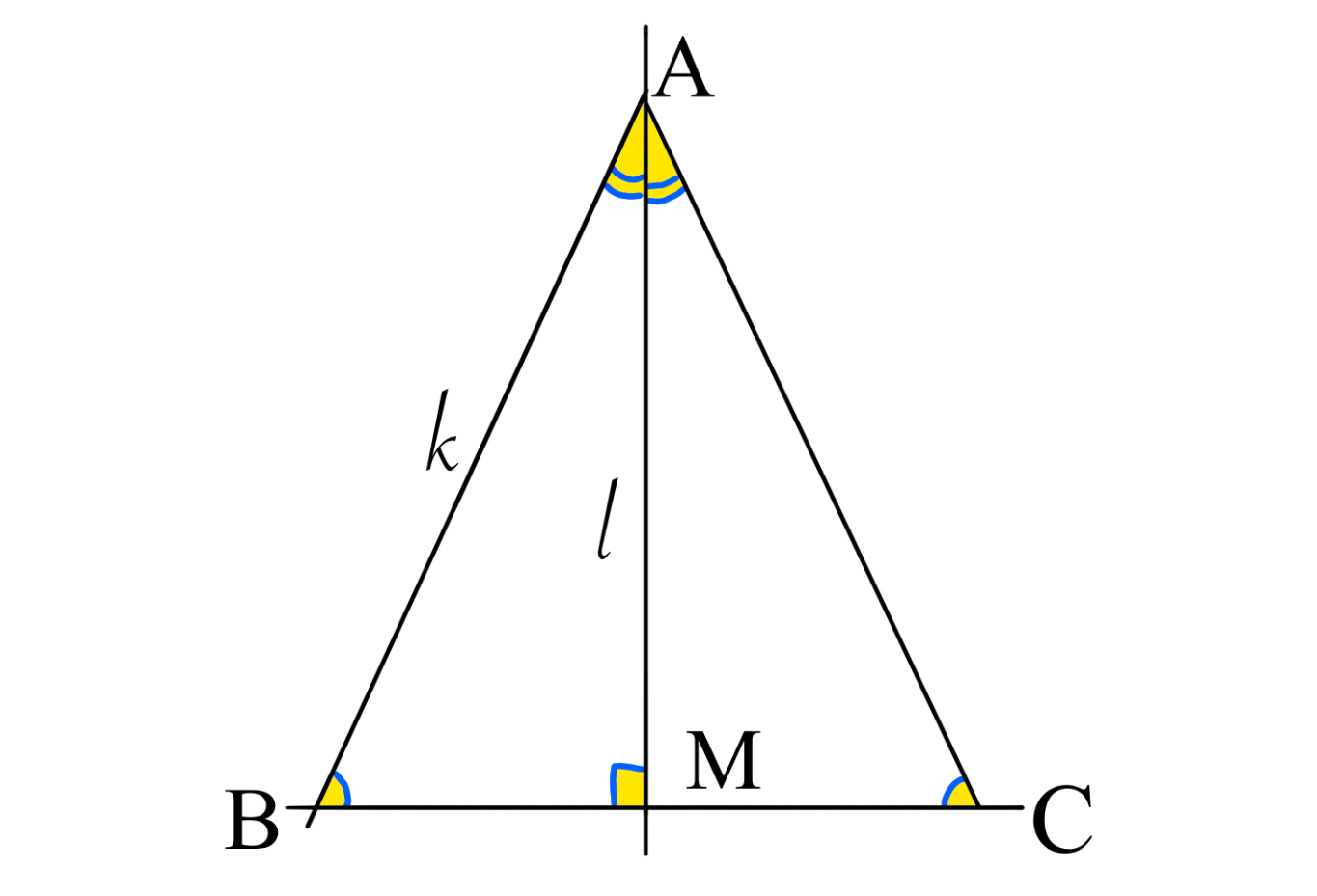Since $\triangle ABC$ is isosceles, $\angle AMB$ is a right angle.
$\Rightarrow \triangle AMB$ is a right angled triangle.
This part uses basic definitions of sin & cos.
Call the length of the hypotenuse of $Rt\triangle AMB$ $k$. Call the length of median $AM$ $l$.
$\Rightarrow \angle BAM=\cos^{-1} \frac{l}{k}.$
$\Rightarrow \angle BAC=2\angle BAM=2\cos^{-1} \frac{l}{k}.$
Since $\triangle AMB$ is right angled, and $\triangle ABC$ is isosceles,
$\sin \angle B=\sin \angle C=\frac{l}{k}.$
$\Rightarrow \angle B=\angle C=\sin^{-1} \frac{l}{k}.$
Let$\frac{l}{k}=n.$.
Finally, since $\angle A,\angle B,\angle C$ are the interior angles of a triangle, i.e. they add up to $\pi (rad)$, $2\cos^{-1} n+2 \sin^{-1} n=\pi$ in radians.

- 3 weeks, 6 days ago

I think this is a new identity that has never been discovered ago?

- 3 weeks, 6 days ago

Maybe, but if so, you're a mathematician officially.

- 3 weeks, 6 days ago

OMG :P

- 3 weeks, 6 days ago

lol, yes OMG

- 3 weeks, 6 days ago

Thx for deleting the comments :)

- 3 weeks, 6 days ago

No prob, sorry bout the notifs

- 3 weeks, 6 days ago

Notifications View all (97 not shown) ...

- 3 weeks, 6 days ago

- 3 months, 2 weeks ago

You can add one more rule: use buttons to decrease length. :)

- 3 months, 2 weeks ago

[[show-hide-button|H]]
[[start-hidden]]
my proof
[[end-hidden]]

- 3 months, 2 weeks ago

Whup!

- 3 months, 2 weeks ago

@Jeff Giff - Buttons don't work in chat, only in notes and wikis, not even problems :)

- 3 months, 2 weeks ago

You can use buttons only in notes :)

- 3 months, 2 weeks ago